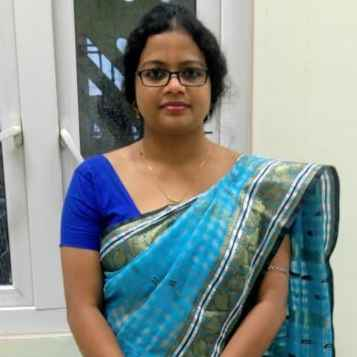X
Projection methods for approximate solution of a class of nonlinear Fredholm integro-differential equations
A. Kayal, G. Nelakanti
Published in Elsevier B.V.
2023
Volume: 184

Pages: 49 - 76
Abstract
The aim of this article is to find the approximate solution of the nonlinear Fredholm integro-differential equations of second kind with smooth kernels with less computational complexity and investigate the asymptotic behavior of convergence of the approximate solutions by using global polynomials based projection methods. We develop the theoretical framework for the nonlinear Fredholm integro-differential equations to obtain the superconvergence results by Legendre polynomial based projection methods and their iterated versions. Numerical examples are considered to demonstrate the theoretical results. © 2022 IMACS
•